## GMAT Remainder Problems: 3 Key TipsIf you’re like me, you probably haven’t thought about remainders in over ten years, when you first learned about long division in elementary school. Remember those lessons on what we call the number that’s left over in a division problem? Yeah, that number’s called a remainder.

And guess what? Remainders show up a lot on the GMAT. Of course, the remainder problems you’ll encounter on the GMAT are much different than the ones you worked on when you were ten years old. GMAT remainder questions can often be quite tricky, but don’t worry! In this guide, I’ll give you a comprehensive overview of GMAT remainder problems so that you feel ready to solve them when you see them on test day.

## The 5 Hardest GMAT Data Sufficiency QuestionsFor many GMAT test takers, Data Sufficiency questions are the most difficult questions on the GMAT. But what do the hardest GMAT Data Sufficiency questions look like? What skills and concepts do they test? What do they have in common? What Data Sufficiency strategies can we use to get these challenging GMAT Quantitative questions right?

In this article, I’ll go over the five hardest GMAT Data Sufficiency questions, what you’ll need to know to solve them, how to approach them on test day, and what we can learn from hard GMAT Quant questions about mastering Data Sufficiency.

## GMAT Exponents: Rules, Tips, and Practice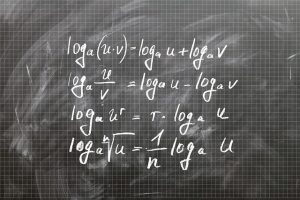Exponents are one of the more frequently tested concepts on the GMAT. It’s likely that you learned all the exponent formulas that you’ll need for the GMAT way back in middle and early high school math, so you’re probably overdue for a refresher! And even if you consider yourself an “exponent expert,” you’re going to have to apply your knowledge of exponents in extremely particular ways on the GMAT, as exponents can appear in a wide variety of question types and are often combined with other mathematical concepts.

Luckily, we’ve done the hard work of distilling everything you need to know about GMAT exponents! In this post, we’ll cover all the relevant rules, properties, formulas, and shortcuts. We’ll also walk you through an example of every main kind of exponent question that you’ll encounter on the test, so you can see these formulas in action. By the end, you really will be an exponent expert!

## GMAT Statistics and Standard Deviation Questions: 4 Key Tips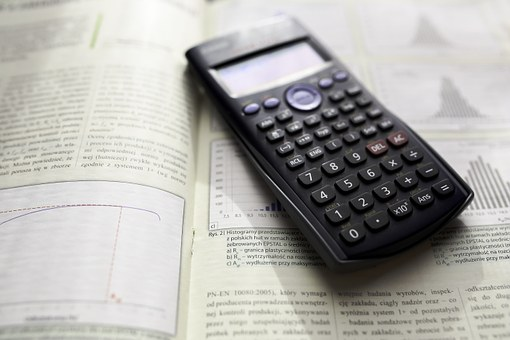If Hayley takes the GMAT three times and scores 750, 770, and 800, respectively, what is her mean score? You probably won’t get a statistics question that’s quite that easy on the GMAT, but the good news about GMAT statistics questions is that they test mostly simple concepts. You don’t need to know any complicated formulas or equations to master statistics for GMAT quant questions, but you do need to develop a solid understanding of descriptive statistics principles.

In this article, I’ll give you an overview of what’s tested in GMAT statistics questions, define the four key definitions you need to know, and give you tips for mastering statistics questions. I’ll also walk you through two GMAT statistics sample questions. And, as an added bonus, I’ll offer up a range of terrible statistics puns. (Get it?) By the end of this article, you’ll have mastered statistics for GMAT!
Continue reading “GMAT Statistics and Standard Deviation Questions: 4 Key Tips”

## GMAT Math Tricks: The 9 Best Tips and Shortcuts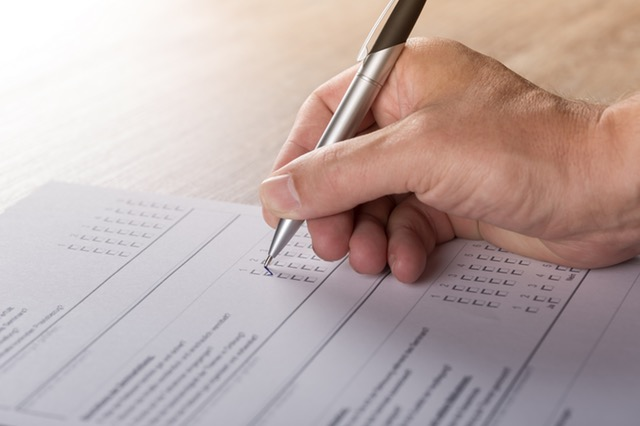As you may already know, the math required for the GMAT Quant section is actually fairly basic: nothing beyond early high school-level math is tested. What’s challenging is how quickly you’ll need to be able to execute calculations to finish within the 62 minutes allotted for the 31 questions and the reasoning and analysis required to get to the right answer. In other words, the GMAT Quant section, like all other sections of the test, is more a test of how you think than what you know.

Luckily, this means that there are several GMAT math tricks, tips, and shortcuts that you can use to improve your performance. In this post, we’ll give you all the major GMAT quant tricks, including tips and shortcuts for each of the two question types as well as some that apply to both. With these GMAT math tricks in your arsenal—plus the boatloads of studying you’re surely doing—you’ll be well prepared to nail the Quant section on test day.
Continue reading “GMAT Math Tricks: The 9 Best Tips and Shortcuts”

## 7 Expert GMAT Data Sufficiency Tips + Examples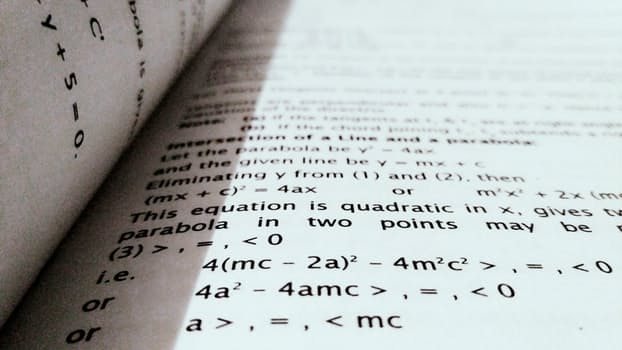Let’s start with the obvious: the data sufficiency questions on the GMAT are really weird. They look nothing like any question you’ve seen in math class. For one thing, every single data sufficiency question has the same five answers. For another, data sufficiency questions aren’t just testing your math skills. They’re also testing your ability to analyze a set of data and decide what information you need to answer the question.

In this guide, I’ll walk you through the GMAT data sufficiency section, give you several strategies for solving GMAT data sufficiency questions, and offer tips for preparing for these unique questions. I’ll also take you through solving several retired sample GMAT data sufficiency questions.

## Every GMAT Geometry Formula You Need to Know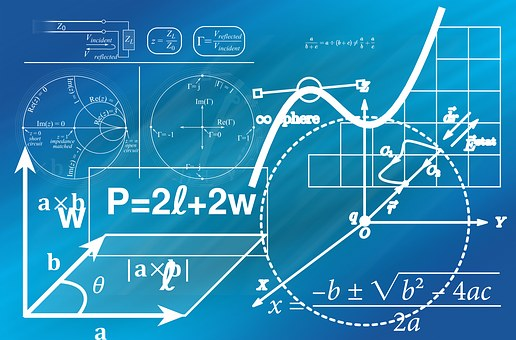If you’re like me, you probably spent a lot of time in high school memorizing the difference between sine and cosine and sighing over long, multi-step proofs, only to forget all of this hard-earned knowledge the second that classes dismissed for break.

If you’ve forgotten a lot of your high school geometry rules or are just in need of a refresher before taking the GMAT, then you’ve found the right article. In this article, I’ll be giving you a comprehensive overview of GMAT geometry.

First, I’ll talk about what and how much geometry is actually on the GMAT. Next, I’ll give you an overview of the most important GMAT geometry formulas and rules you need to know. Then, I’ll show you four geometry sample questions and explain how to solve them. Finally, I’ll talk about how to study for the geometry you’ll encounter on the GMAT and give you tips for acing test day.

## GMAT Rate Problems: How to Conquer All 3 TypesTwo cars bound for the same GMAT test center leave their houses at the exact same time. Car A travels 30 miles per hour; Car B travels 20 miles per hour. Car A has to travel 20 miles. Car B has to travel 15 miles. Which car will arrive first?